Publication date: 03/23/2021

## Ordinal Responses

With an ordinal response (Y), as with nominal responses, JMP fits probabilities that the response is one of r different response levels given by the data.

Ordinal data have an order like continuous data. The order is used in the analysis but the spacing or distance between the ordered levels is not used. If you have a numeric response but want your model to ignore the spacing of the values, you can assign the ordinal level to that response column. If you have a classification variable and the levels are in some natural order such as low, medium, and high, you can use the ordinal modeling type.

Ordinal responses are modeled by fitting a series of parallel logistic curves to the cumulative probabilities. Each curve has the same design parameters but a different intercept and is specified as follows: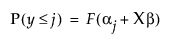for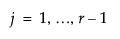where r response levels are present and F(x) is the standard logistic cumulative distribution function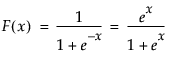Another way to write this is in terms of an unobserved continuous variable, z, that causes the ordinal response to change as it crosses various thresholds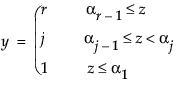where z is an unobservable function of the linear model and error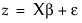and ε has the logistic distribution.

These models are attractive because they recognize the ordinal character of the response, they need far fewer parameters than nominal models, and the computations are fast.

A different but mathematically equivalent way to envision an ordinal model is to think of a nominal model where, instead of modeling the odds, you model the cumulative probability. Instead of fitting functions for all but the last level, you fit only one function and slide it to fit each cumulative response probability.

### Fitting Principle for Ordinal Response

The maximum likelihood fitting principle for an ordinal response model is the same as for a nominal response model. It estimates the parameters such that the joint probability for all the responses that occur is the greatest obtainable by the model. It uses an iterative method that is faster and uses less memory than nominal fitting.

### Base Model

The simplest model for an ordinal response, like a nominal response, is a set of response probabilities fitted as the occurrence rates of the response in the whole data table.

Want more information? Have questions? Get answers in the JMP User Community (community.jmp.com).